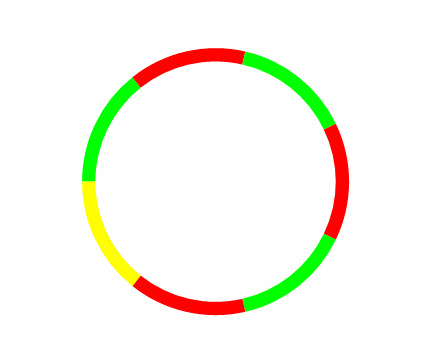PowerPoint tips, hints and tutorials that will change your presentations for ever!

INDEXPowerPoint vba Segmented Ring

Suppose you want to make a ring shape split into a number of segments and need to individually fill each one.Not that simple?! This code will do it for you.

DON'T KNOW HOW TO USE CODE??

Sub makeSegments()
Dim osld As Slide
Dim oshp() As Shape
Dim i As Integer
Dim icount As Integer
Dim sngAngle As Single
On Error Resume Next
Set osld = ActiveWindow.View.Slide
If osld Is Nothing Then
MsgBox "No slide selected!", vbCritical
Exit Sub
End If
If ActiveWindow.Selection.ShapeRange.Count > 0 Then ActiveWindow.Selection.Unselect
icount = InputBox("How many segments")
sngAngle = 360 / icount
ReDim oshp(1 To 1)
For i = 1 To icount
Left:=100, _
Top:=100, _
Width:=200, _
Height:=200)
oshp(i).Line.Visible = msoFalse
If i / 2 = i \ 2 Then oshp(i).Fill.ForeColor.RGB = vbRed Else _
oshp(i).Fill.ForeColor.RGB = vbGreen
oshp(i).Adjustments(1) = 180 + ((i - 1) * sngAngle)
If oshp(i).Adjustments(1) > 360 Then _
If oshp(i).Adjustments(2) > 360 Then _
oshp(i).Select (msoFalse)
ReDim Preserve oshp(1 To UBound(oshp) + 1)
If i = icount And icount / 2 <> icount \ 2 Then _
oshp(i).Fill.ForeColor.RGB = vbYellow
Next
ActiveWindow.Selection.ShapeRange.Group
End Sub

If you need to adjust the Thickness of the ring you can change the value of Adjustment(3) from 0.05 to a value between 0.5  and 0.01.

The shape is group so it can be easily resized but if you wish to animate the segments or recolor you can of course ungroup it.

Back to the Index Page